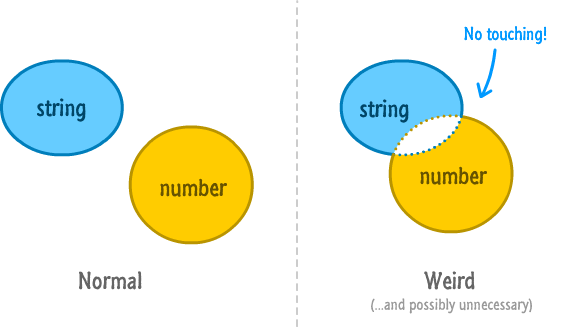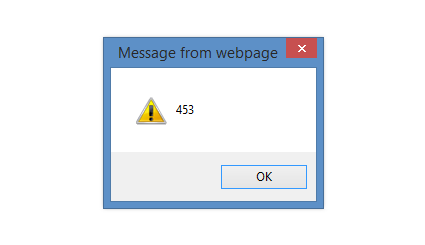Color

Background

Done

# Going from a String to a Number

by kirupa   |   filed under JavaScript 101

By design, strings deal with text-based things. Numbers deal with...um...numbers. For the most part, unless you are displaying some text that includes numerical data, you will never need to mix and match numbers and strings together. That would just be weird and possibly unnecessary:Of course, this wouldn't be HTML if you didn't find yourself doing unnecessary or weird things all the time. There are many cases where something that should be a number is actually returned to you as a string. If you've ever worked with data returned from a web service, fiddled with setting or getting CSS property values, or fell into any other situation where the data you are working with isn't entirely under your control, you totally know what I am talking about.

In this short tutorial, you will learn some simple tricks on how to convert a string into a number so that you can do all sorts of number-ish things to it.

Onwards!

## Meet parseInt

Let's start with a very contrived situation that drives the point across:

```var numberOne = "45";
var numberTwo = 3;

What do you think is going to be displayed when you add numberOne and numberTwo? Well, here is what gets displayed:This probably isn't what you wanted. This is because numberOne is a string and numberTwo is a number. In the world of JavaScript, the result of adding a string to a number is a string. What seems like a straightforward mathematical operation to us is a bizarre string addition (aka concatentation...as the cool kids say) to your browser. The scary part is how subtle this is.

The way you can fix this is by never finding yourself in this situation in the first place. When that isn't possible, you should convert the string into a number before you perform your addition. That is surprisingly easy to do thanks to the parseInt function. Below is a version of the above code where I revised our example to use parseInt to convert the 45 into a number before getting into the mathematical expression:

```var numberOne = parseInt("45");
var numberTwo = 3;

This time around, because both numberOne (thanks to parseInt) and numberTwo are numbers, the value you will see is 48.

Now, if the numerical string you are dealing with contains decimals, parseInt will convert that into an integer-based number. This means if you used parseInt on, let's say, 2.99, you will see a 2 as a result.  You probably don't want that. To help you preserve those extra digits of precision, you have the parseFloat function which works very similar to parseInt:

```var itemOne = parseFloat("2.99");
var itemTwo = 4;
var total = 1.08 * (itemOne + itemTwo);

To use parseFloat, simply pass in the string that you want converted into a number whose representation is a float.

## More Realistic Example Involving CSS Properties

Now that you've seen how to use parseInt and parseFloat using a pretty silly example, let's kick things up a few notches by looking at a situation where I had to use parseInt in real life!

Let's say you have an element with an id of mySquare whose width is set by the following style rule:

```#mySquare {
width: 250px;
height: 250px;
background-color: green;
}```

What I wanted to do is increase the width of this element by 10 pixels. To handle that, here is the code that I had initially:

```var myElement = document.querySelector("#mySquare");
var style = window.getComputedStyle(myElement);

// getting 250 and removing the "px" part of the value
var initialWidth = style.getPropertyValue("width").split("px");

// adding 10 and tagging "px" back
var newWidth = initialWidth + 10 + "px";
myElement.style.width = newWidth;

Can you guess what the value for newWidth would be in the end?

The answer wasn't 260px as I would have wanted. Instead, the answer turned out to be 25010px instead. The problem lies in the following line:

```var myElement = document.querySelector("#mySquare");
var style = window.getComputedStyle(myElement);

// getting 250 and removing the "px" part of the value
var initialWidth = style.getPropertyValue("width").split("px");

// adding 10 and tagging "px" back
var newWidth = initialWidth + 10 + "px";
myElement.style.width = newWidth;

The value of initialWidth at this point is 250...in the form of a string! When I attempt to add 10 to this value, what you get is the equivalent of combining two strings together: 25010. The solution is to wrap the initialWidth variable into a parseInt function before using it as part of my addition:

```var myElement = document.querySelector("#mySquare");
var style = window.getComputedStyle(myElement);

// getting 250 and removing the "px" part of the value
var initialWidth = style.getPropertyValue("width").split("px");

// adding 10 and tagging "px" back
var newWidth = parseInt(initialWidth) + 10 + "px";
myElement.style.width = newWidth;

Once I did this, the value for newWidth was what I expected it to be at 260. You can see another example of me using the parseInt function in an example where I display PHP data in a HTML page.

## Wait, There's More!

If you thought the story with parseInt and parseFloat ended, then you are in for a surprise. These two functions along with some JavaScript quirks result in some pretty strange behavior that can only be explained by looking at examples:

```// NaN
parseInt("foo");

// 200
parseInt("200 + 400 + 300");

// 200
parseInt("200abc")

// NaN
parseInt("abc200");

// 155
"15" + 5;

// 3
"15" / 5;

// 65
"15" * 5;

// 4
parseInt("4.55");```

Some of the results make sense. Some of the results are a bit strange...like dividing or multiplying stringified numbers ends up converting the string into a number first. That is different from what you see with the + operator.

#### The Number Function

Besides parseInt and parseFloat, you also have the Number function that you can use to turn a string into a number:

`Number("3.14") // 3.14 as a number`

For normal numbers that don't involve any strings or other shenanigans, the the results you get with the Number function is identical to what you will see using parseInt or parseFloat. If the string you are trying to convert contains non-numerical data such as letters, then the Number function will fail:

`Number("20px") // NaN`

For this example, both parseInt and parseFloat will capture and return the 20 from the string. The Number function will return nothing. Which you want to use depends entirely on your scenario, but I often end up using parseFloat since the strings I convert often include text-based data.

## Conclusion

JavaScript is a bit of a fuzzy language when it comes to clearly defining what you can and can't do when you put a string and a number together in the same room. Jumping between a string to a number (or vice-versa) is not a cheap operation. If you care about performance, you want to minimize how often you cross the string/number divide.

Thanks also to Axel Rauschmayer for reminding me about the Number function!

Just a final word before we wrap up. If you have a question and/or want to be part of a friendly, collaborative community of over 220k other developers like yourself, post on the forums for a quick response!